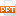# Bài giảng môn học Quản trị kinh doanh - Chapter three: Decimals

LU 3-1: Rounding Decimals; Fraction & Decimal Conversions

Explain the place values of whole numbers and decimals; round decimals.

Convert decimal fractions to decimals, proper fractions to decimals, mixed numbers to decimals, and pure and
mixed decimals to decimal fractions.

LU 3-2: Adding, Subtracting, Multiplying, and Dividing Decimals

Add, subtract, multiply, and divide decimals.

Complete decimal applications in foreign currency.

Multiply and divide decimals by shortcut methods.18 trang | Chia sẻ: hongha80 | Lượt xem: 200 | Lượt tải: 1
Nội dung tài liệu Bài giảng môn học Quản trị kinh doanh - Chapter three: Decimals, để tải tài liệu về máy bạn click vào nút DOWNLOAD ở trên
•spptchap003_7041.pptx